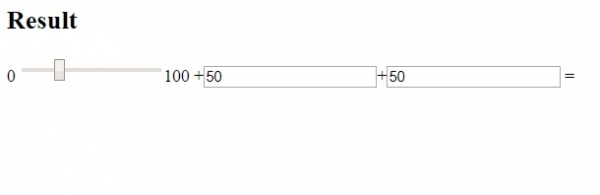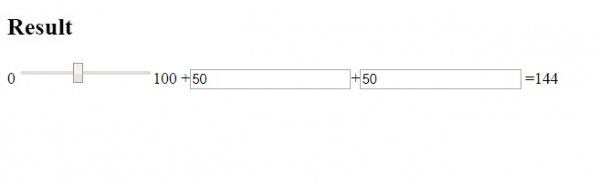# HTML Tag

The <output> tag in HTML is used to display the output of a calculation. It introduced in HTML5.

Following are the attributes −

• for - Relationship between the result of the calculation and the elements used in the calculation
• form - Forms the output element belongs to
• name - Name for the output element

Let us now see an example to implement the <output> tag −

## Example

Live Demo

<!DOCTYPE html>
<html>
<body>
<h2>Result</h2>
<form oninput="n.value=parseInt(p
.value)+parseInt(q.value)+parseInt(r.value)">0
<input type="range" id="p" value="25">100
+<input type="number" id="q" value="50">+<input type="number" id="r" value="50">
=<output name="n" for="p q r"></output>
</form>
</body>
</html>

## OutputNow, increase the slider and the result would get displayed −Updated on: 11-Jun-2020

62 Views